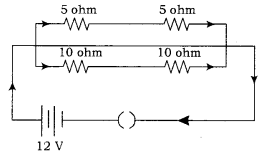# If a 12V'battery is connected to the arrangement of resistances given below; calculate: (i) the total effective resistance of the arrangement

If a 12V’battery is connected to the arrangement of resistances given below; calculate:
(i) the total effective resistance of the arrangement and,
(ii) the total current flowing in the circuit.

(i) The 5Ω resistors are connected in series.
Therefore, their effective resistance = (5 +5) = 1OΩ
The 10Ω resistors are connected in series.
Therefore, their effective resistance = (10 + 10) = 20ΩNow, these 10Ω equivalent and 20Ω equivalent are connected in parallel. Therefore,
the equivalent resistance (Req) will be(ii)Total current = Total Voltage/Total equivalent resistance
= 12/6.67= 1.8A